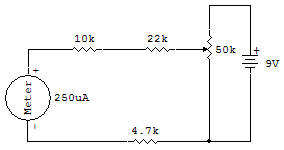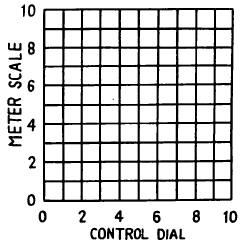Diagram trace of a variable resistorThe main purpose of this project is to study the characteristics of the 50K Control with a voltmeter circuit and then graph the results. The purpose of a potentiometer is to provide a variable control of an output voltage (between the center connection and one end of the Control).

The arrow head on the Schematic symbol of the Control tells you it is adjustable. This variable output voltage is measured with a voltmeter. The 9V Battery is used as the constant voltage across the entire potentiometer. The DC voltmeter used for voltage measurements is described more completely in another project on DC voltmeters, so we'll just give you a brief description here.

The Meter requires about 250 microamperes for a full scale deflection. Therefore, a series resistance totaling 36.7K ohms (10K, 22K and 4.7K) is used with the Meter. This provides a Meter circuit which requires about 9.3V to obtain a full scale reading.

This Meter then is capable of measuring all potentiometer output voltages up to the (9V of the Battery). The blue scale on the Meter is used for this circuit because these calibrations give accurate results in terms of actual DC voltages.

1. Set the Control at zero and record the Meter blue scale reading by placing a dot on the graph provided below.

2. Set the Control at 1 and record the Meter's blue scale reading with a second dot on the graph.

3. Repeat the above procedure for each Control number setting.

4. Sketch in a smooth line connecting all the dots together. The result is the graph of relative potentiometer output voltage when loaded with a load of about 37K ohms.

The potentiometer in this circuit typically has a smooth change in output between about 1 and 9 on the dial. The change in output is nearly a straight line. This straight line output is characteristic of a LINEAR potentiometer.

Other, potentiometers are available with other than a straight line output. Some common types are the LOG, SEMI-LOG and REVERSE LOG tapers.Recherche personnalisée